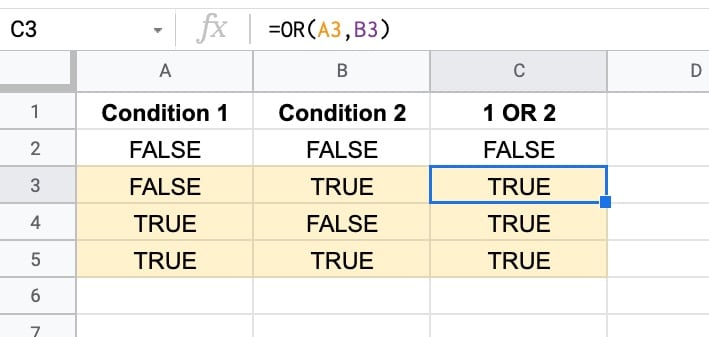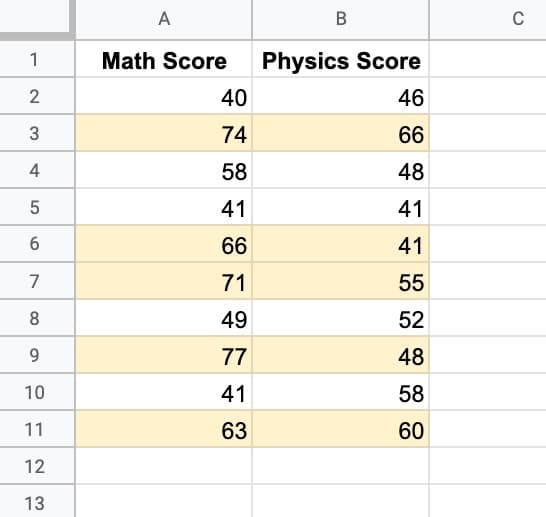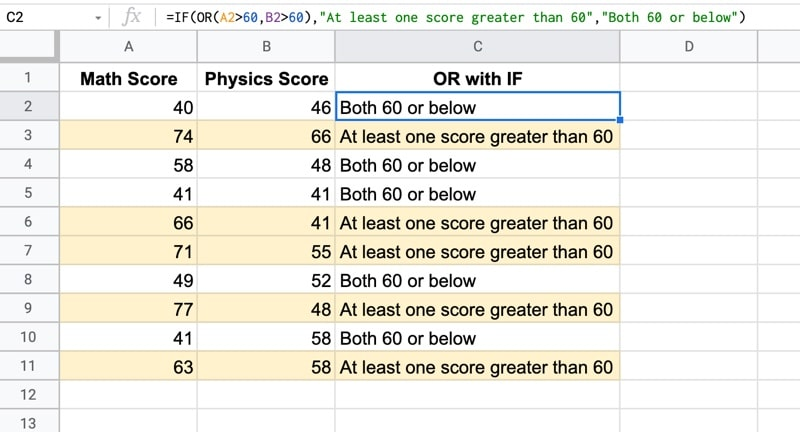# The OR Function in Google Sheets

The OR function in Google Sheets is a logical function that outputs TRUE if at least one of the inputs is TRUE, and FALSE only if all the inputs are FALSE.It has useful applications in data cleaning where it pairs well with the IF Function and AND Function to categorize and organize data.

## OR Formula Example

Suppose we have the following dataset, showing scores across two exams:We want to find all the students who scored more than 60 in at least one of the exams. In other words, they scored more than 60 in Math or they scored more than 60 in Physics. (I’ve highlighted the rows that meet these criteria in yellow.)

So the first logical test, in cell C2, is:

`= A2 > 60`

And the second logical test, in cell D2, is:

`= B2 > 60`

The results of these two test expressions can then be fed into an OR formula:

`=OR(C2:D2)`

or

`=OR(C2,D2)`

Both of these OR formulas produce the same result. You can enter ranges or cells of data.

We can also drop the two expressions directly into the OR formula, like this:

`=OR(A2 > 60 , B2 > 60)`

All of these OR formulas will output TRUE when either or both of the inputs are TRUE, otherwise, the output will be FALSE.## OR Function Syntax

`=OR(logical_expression1, [logical_expression2, ...])`

It takes one or more arguments, which can be cells, ranges, or expressions containing logical tests.

It’s part of the Logical family of functions in Google Sheets, along with the AND function.

### Function Notes

Let’s look at the OR function inputs more closely:

Expression Inputs

Expression inputs are tests that result in TRUE or FALSE values.

For example, here are some expressions that output a TRUE or FALSE result and can therefore be inserted into an OR formula.

`A1 = "Some Value"`
`B1 > 100`
`ISEMAIL(A1)`
`A1 < B1`
`ISEVEN(A1)`

These expressions can be in their own cells and referenced in the OR formula like this:

`=OR(C1,D1)`

or they can be dropped directly into OR like this:

`=OR(A1 = "Some Value",B1 > 100)`

Number Inputs

0 is interpreted as FALSE by the OR Function. All other numbers are interpreted as TRUE.

So `OR(1,1)` is TRUE, `OR(1,0)` is TRUE, but `OR(0,0)` is FALSE.

Text Inputs

Generally ignored by OR functions although there is some nuance around this. See this Twitter thread (it's based on the AND function but it's the same principle).

## OR Formula Template

Feel free to make a copy: File > Make a copy...

If you can't access the template, it might be because of your organization's Google Workspace settings. If you right-click the link and open it in an Incognito window you'll be able to see it.

## OR + IF Function

OR pairs nicely with the IF Function because the TRUE/FALSE output from the OR formula feeds directly into the first argument of the IF function.

Using the same example as earlier, suppose we want to label all the students who score more than 60 in at least one of the exams.

We simply put the OR formula from earlier into the first argument of our IF function:

`=IF(OR(A2 > 60 , B2 > 60) , "At least one score greater than 60" , "Both 60 or below" )`

The output of this formula is: